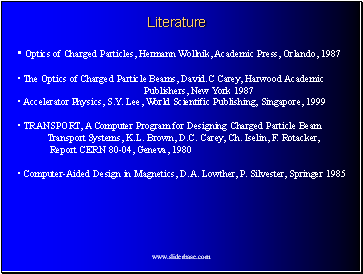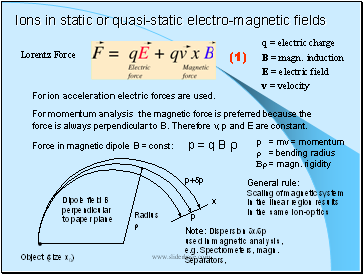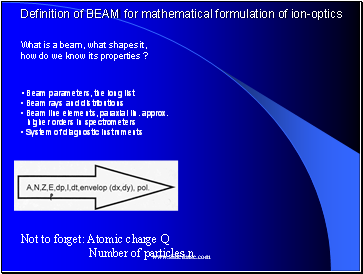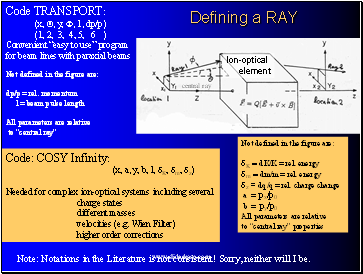# An Introduction to Ion-OpticsPage 2

#### WATCH ALL SLIDES

Linear Algebra (Matrix calculations), first order ion-optics (e.g. TRANSPORT)

Higher order ion-optics code to solve equation of motion, (e.g. COSY Infinity,

GIOS, RAYTRACE (historic)

Electro-magnetic field program (solution of Maxwell’s Equations),

(e.g. finite element (FE) codes, 2d & 3d: POISSON, TOSCA, MagNet)

Properties of incoming charged particles and design function of electro-magnetic

facility, beam, reaction products (e.g. kinematic codes, charge distributions of

heavy ions, energy losses in targets)

Many other specialized programs, e.g for accelerator design (e.g. synchrotrons,

cyclotrons) not covered in this lecure series.

Slide 7## Literature

Optics of Charged Particles, Hermann Wollnik, Academic Press, Orlando, 1987

The Optics of Charged Particle Beams, David.C Carey, Harwood Academic

Publishers, New York 1987

Accelerator Physics, S.Y. Lee, World Scientific Publishing, Singapore, 1999

TRANSPORT, A Computer Program for Designing Charged Particle Beam

Transport Systems, K.L. Brown, D.C. Carey, Ch. Iselin, F. Rotacker,

Report CERN 80-04, Geneva, 1980

Slide 8Ions in static or quasi-static electro-magnetic fields

Lorentz Force

q = electric charge

B = magn. induction

E = electric field

v = velocity

For momentum analysis the magnetic force is preferred because the force is always perpendicular to B. Therefore v, p and E are constant.

Force in magnetic dipole B = const: p = q B r

p = mv = momentum

Br = magn. rigidity

For ion acceleration electric forces are used.

Dipole field B

perpendicular

to paper plane

Object (size x0)

General rule:

Scaling of magnetic system

in the linear region results

in the same ion-optics

Note: Dispersion dx/dp

used in magnetic analysis,

e.g. Spectrometers, magn.

Separators,

x

p

p+dp

(1)

Slide 9Definition of BEAM for mathematical formulation of ion-optics

What is a beam, what shapes it,

how do we know its properties ?

Beam parameters, the long list

Beam rays and distributions

Beam line elements, paraxial lin. approx.

higher orders in spectrometers

System of diagnostic instruments

Not to forget: Atomic charge Q

Number of particles n

Slide 10## Defining a RAY

Ion-optical

element

Code TRANSPORT:

(x, Q, y, F, 1, dp/p)

(1, 2, 3, 4, 5, 6 )

Convenient “easy to use” program

Go to page:
1  2  3  4  5  6